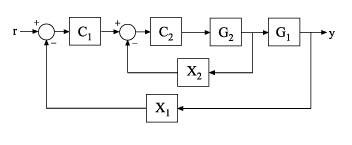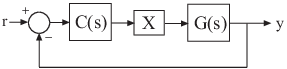# getLoopTransfer

Open-loop transfer function of control system represented by `genss` model

## Syntax

``L = getLoopTransfer(T,Locations)``
``L = getLoopTransfer(T,Locations,sign)``
``L = getLoopTransfer(T,Locations,sign,openings)``

## Description

example

````L = getLoopTransfer(T,Locations)` returns the point-to-point open-loop transfer function of a control system at specified analysis points. The control system is represented by a generalized state-space model `T`, containing the analysis points specified by `Locations`. The point-to-point open-loop transfer function is the response obtained by opening the loop at the specified locations, injecting signals at those locations, and measuring the return signals at the same locations. ```

example

````L = getLoopTransfer(T,Locations,sign)` specifies the feedback sign for calculating the open-loop response. The relationship between the closed-loop response `T` and the open-loop response `L` is `T = feedback(L,1,sign)`.```

example

````L = getLoopTransfer(T,Locations,sign,openings)` specifies additional loop-opening locations to open for computing the open-loop response at `Locations`. ```

## Examples

collapse all

Compute the open-loop response of the following control system model at an analysis point specified by an `AnalysisPoint` block, `X`.Create a model of the system by specifying and connecting a numeric LTI plant model, `G`, a tunable controller, `C`, and the `AnalysisPoint`, `X`.

```G = tf([1 2],[1 0.2 10]); C = tunablePID('C','pi'); X = AnalysisPoint('X'); T = feedback(G*X*C,1);```

`T` is a `genss` model that represents the closed-loop response of the control system from $r$ to $y$. The model contains `AnalysisPoint` block `X`, which identifies the potential loop-opening location.

Calculate the open-loop point-to-point loop transfer at location `X`.

`L = getLoopTransfer(T,'X');`

This command computes the transfer function you would obtain by opening the loop at `X`, injecting a signal into `G`, and measuring the resulting response at the output of `C`. By default, `getLoopTransfer` computes the positive feedback transfer function, which is the loop transfer assuming that the loop will be closed at `X` without a change of sign. In this example, the positive feedback transfer function is $L\left(s\right)=-C\left(s\right)G\left(s\right)$.

The output `L` is a `genss` model that includes the tunable block `C`. You can use `getValue` to obtain the current value of `L`, in which all the tunable blocks of `L` are evaluated to their current numeric value.

Compute the stability margins of the following closed-loop system at an analysis point specified by an `AnalysisPoint` block, `X`.Create a model of the system by specifying and connecting a numeric LTI plant model `G`, a tunable controller `C`, and the `AnalysisPoint` block `X`.

```G = tf([1 2],[1 0.2 10]); C = pid(0.1,1.5); X = AnalysisPoint('X'); T = feedback(G*X*C,1);```

`T` is a `genss` model that represents the closed-loop response of the control system from $r$ to $y$. The model contains the `AnalysisPoint` block `X` that identifies the potential loop-opening location.

By default, `getLoopTransfer` returns a transfer function `L` at the specified analysis point such that `T = feedback(L,1,+1)`. However, `margin` assumes negative feedback, so that `margin(L)` computes the stability margin of the negative feedback closed-loop system `feedback(L,1)`. Therefore, to analyze the stability margins, set the `sign` input argument to `-1` to extract a transfer function `L` such that `T = feedback(L,1)`. In this example, this transfer function is $L\left(s\right)=C\left(s\right)G\left(s\right)$.

`L = getLoopTransfer(T,'X',-1);`

This command computes the open-loop transfer function from the input of `G` to the output of `C`, assuming that the loop is closed with negative feedback, so that you can use it with analysis commands like `margin`.

`[Gm,Pm] = margin(L)`
```Gm = 1.4100 ```
```Pm = 4.9486 ```

Compute the open-loop response of the inner loop of the following cascaded control system, with the outer loop open.Create a model of the system by specifying and connecting the numeric plant models `G1` and `G2`, the tunable controllers `C1`, and the `AnalysisPoint` blocks `X1` and `X2` that mark potential loop-opening locations.

```G1 = tf(10,[1 10]); G2 = tf([1 2],[1 0.2 10]); C1 = tunablePID('C','pi'); C2 = tunableGain('G',1); X1 = AnalysisPoint('X1'); X2 = AnalysisPoint('X2'); T = feedback(G1*feedback(G2*C2,X2)*C1,X1);```

Compute the negative-feedback open-loop response of the inner loop, at the location `X2`, with the outer loop opened at `X1`.

`L = getLoopTransfer(T,'X2',-1,'X1');`

By default, the loop is closed at the analysis-point location marked by the `AnalysisPoint` block `X1`. Specifying `'X1'` for the `openings` argument causes `getLoopTransfer` to open the loop at `X1` for the purposes of computing the requested loop transfer at `X2`. In this example, the negative-feedback open-loop response $L\left(s\right)={G}_{2}\left(s\right){C}_{2}\left(s\right)$.

## Input Arguments

collapse all

Model of a control system, specified as a Generalized State-Space (`genss`) Model. Locations at which you can open loops and perform open-loop analysis are marked by `AnalysisPoint` blocks in `T`.

Analysis-point locations in the control system model at which to compute the open-loop point-to-point response, specified as a character vector or a cell array of character vectors that identify analysis-point locations in `T`.

Analysis-point locations are marked by `AnalysisPoint` blocks in `T`. An `AnalysisPoint` block can have single or multiple channels. The `Location` property of an `AnalysisPoint` block gives names to these feedback channels.

The name of any channel in an `AnalysisPoint` block in `T` is a valid entry for the `Locations` argument to `getLoopTransfer`. To get a full list of available analysis points in `T`, use `getPoints(T)`.

`getLoopTransfer` computes the open-loop response you would obtain by injecting a signal at the implicit input associated with an `AnalysisPoint` channel, and measuring the response at the implicit output associated with the channel. These implicit inputs and outputs are arranged as follows.`L` is the open-loop transfer function from `in` to `out`.

Sign of open-loop transfer function for analysis, specified as `+1` or `-1`.

By default, for an input closed-loop system `T`, the function returns a transfer function `L` at the specified analysis point, such that `T = feedback(L,1,+1)`. However, certain analysis commands that take an open-loop response assume that the loop will be closed with negative feedback. For instance, `margin(L)` computes the stability margin of the negative feedback closed-loop system `feedback(L,1)`. Similarly, the stability margins you can obtain by right-clicking on a `bode` plot make the same assumption. Therefore, when you use `getLoopTransfer` to extract an open-loop transfer function with the purpose of analyzing closed-loop stability, you can set `sign` = `-1` to extract a transfer function `L` such that ```T = feedback(L,1)```.

For example, consider the following system, where `T` is the closed-loop transfer function from r to y.By default, `L = getLoopTransfer(T,'X')` computes the transfer function L = –C(s)G(s), such that `T = feedback(L,1,+1)`. To compute the stability margins at `X` using the `margin` command, which assumes negative feedback, you must compute a transfer function L = C(s)G(s), such that `T = feedback(L,1)`. To do so, use ```L = getLoopTransfer(T,'X',-1)```.

Additional locations for opening feedback loops for computation of the open-loop response, specified as character vector or cell array of character vectors that identify analysis-point locations in `T`. Analysis-point locations are marked by `AnalysisPoint` blocks in `T`. Any channel name contained in the `Location` property of an `AnalysisPoint` block in `T` is a valid entry for `openings`.

Use `openings` when you want to compute the open-loop response at one analysis-point location with other loops also open at other locations. For example, in a cascaded loop configuration, you can calculate the inner loop open-loop response with the outer loop also open. Use `getPoints(T)` to get a full list of available analysis-point locations in `T`.

## Output Arguments

collapse all

Point-to-point open-loop response of the control system `T` measured at the analysis points specified by `Locations`, returned as a generalized state-space (`genss`) model.

• If `Locations` specifies a single analysis point, then `L` is a SISO `genss` model. In this case, `L` represents the response obtained by opening the loop at `Locations`, injecting signals and measuring the return signals at the same location.

• If `Locations` is a vector signal, or specifies multiple analysis points, then `L` is a MIMO `genss` model. In this case, `L` represents the open-loop MIMO response obtained by opening loops at all locations listed in `Locations`, injecting signals and measuring the return signals at those locations.

## Tips

• You can use `getLoopTransfer` to extract open-loop responses given a generalized model of the overall control system. This is useful, for example, for validating open-loop responses of a control system that you tune with the a tuning command such as `systune`.

• `getLoopTransfer` is the `genss` equivalent to the Simulink® Control Design™ command `getLoopTransfer` (Simulink Control Design), which works with the `slTuner` and `slLinearizer` interfaces. Use the Simulink Control Design command when your control system is modeled in Simulink.

Introduced in R2012b